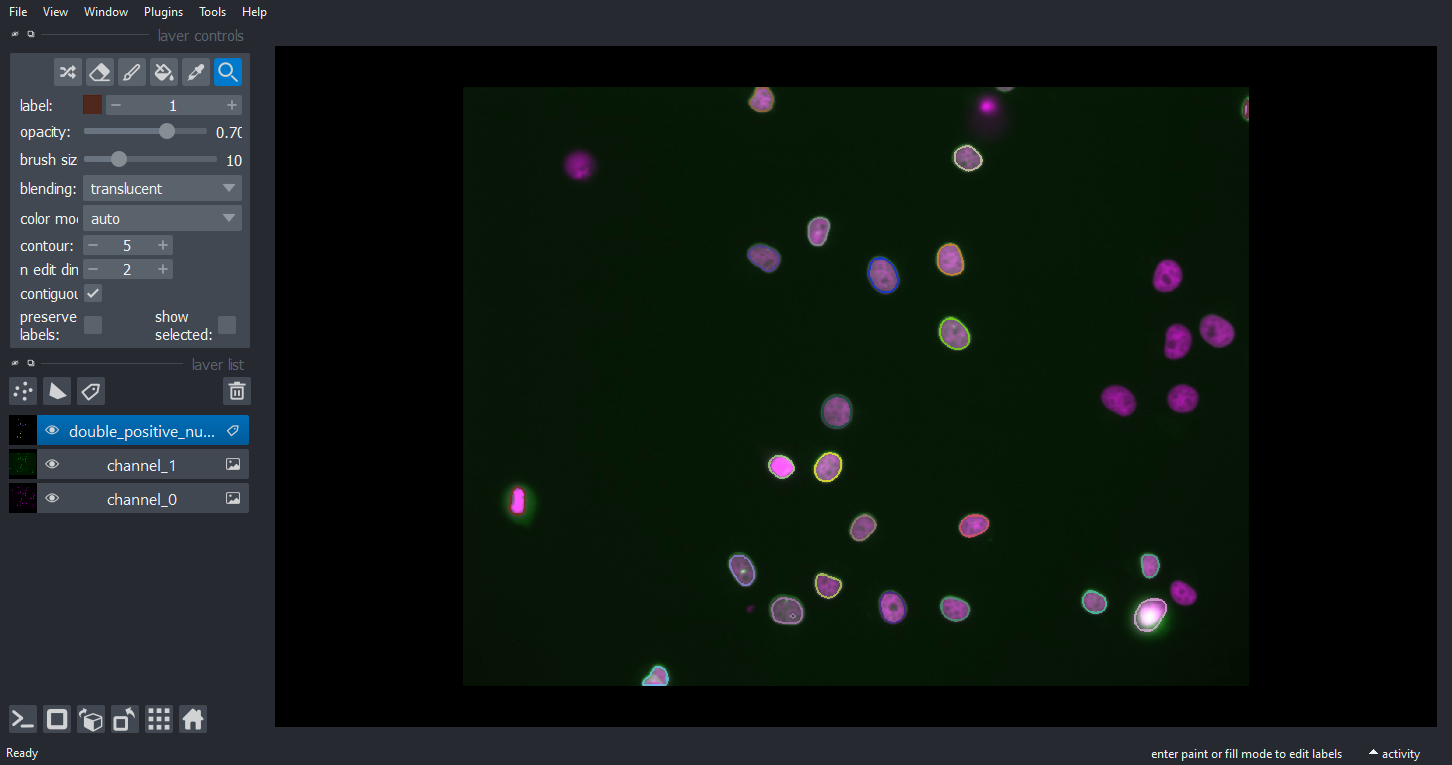# Counting nuclei according to expression in multiple channels#

A common bio-image analysis task is counting cells according to their signal expression in multiple channels. In this example we take a two-channel image of nuclei which express Cy3 and eGFP. Visually, we can easily see that some nuclei expressing Cy3 also express eGFP, others don’t. This notebook demonstrates how to count these groups of nuclei.

import pyclesperanto_prototype as cle
import numpy as np
import matplotlib.pyplot as plt

cle.get_device()

<Intel(R) Iris(R) Xe Graphics on Platform: Intel(R) OpenCL HD Graphics (1 refs)>


We’re using a dataset published by Heriche et al. licensed CC BY 4.0 available in the Image Data Resource.

# load file
raw_image = imread('../../data/plate1_1_013 [Well 5, Field 1 (Spot 5)].png')

# visualize
imshow(raw_image)

<matplotlib.image.AxesImage at 0x2b453c8f700>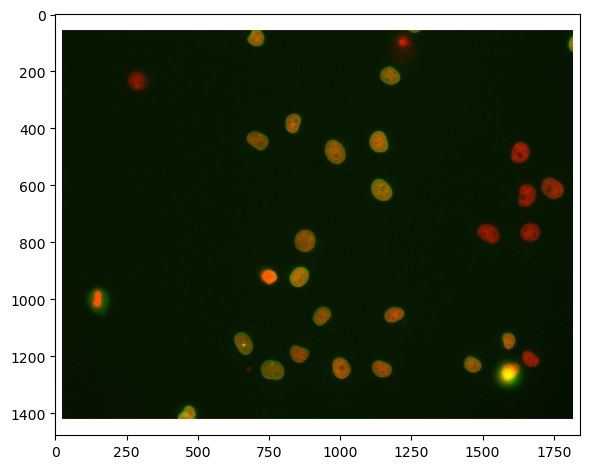First, we need to split channels (read more). After that, we can actually see that not all cells marked with Cy3 (channel 0) are also marked with eGFP (channel 1):

# extract channels
channel_0 = raw_image[...,0]
channel_1 = raw_image[...,1]

# visualize
fig, axs = plt.subplots(1, 2, figsize=(15,15))
axs.imshow(channel_0, cmap='gray')
axs.imshow(channel_1, cmap='gray')

<matplotlib.image.AxesImage at 0x2b453c8f730>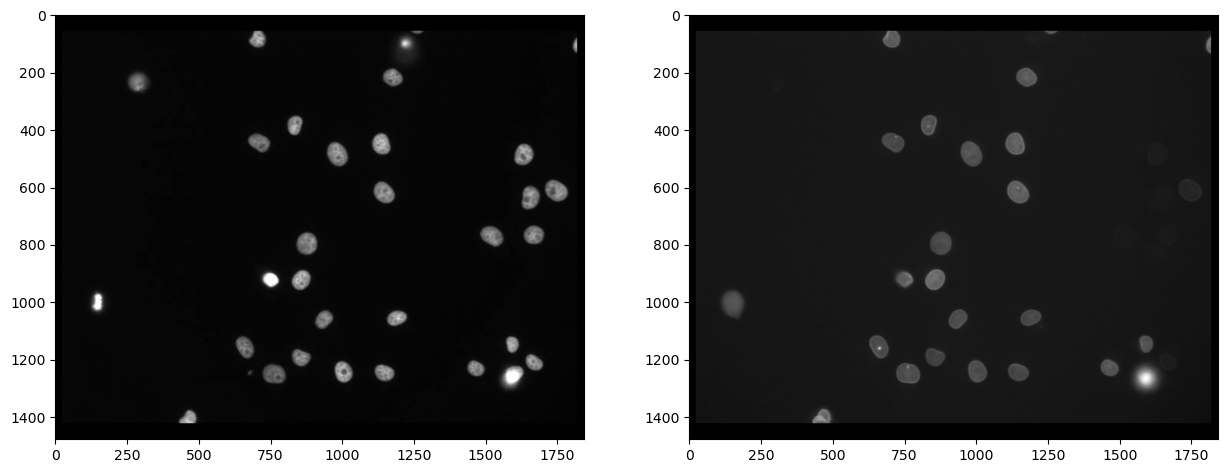## Segmenting nuclei#

As the staining marks the whole nucleus in both cases, it is reasonable to segmentn nuclei in both images and then process the segmented images further. We use Voronoi-Otsu-Labeling for the segmentation because it is a quick and straightforward approach.

# segmentation
nuclei_cy3 = cle.voronoi_otsu_labeling(channel_0, spot_sigma=20)
nuclei_egfp = cle.voronoi_otsu_labeling(channel_1, spot_sigma=20)

# visualize
fig, axs = plt.subplots(1, 2, figsize=(15,15))
cle.imshow(nuclei_cy3, plot=axs, labels=True)
cle.imshow(nuclei_egfp, plot=axs, labels=True)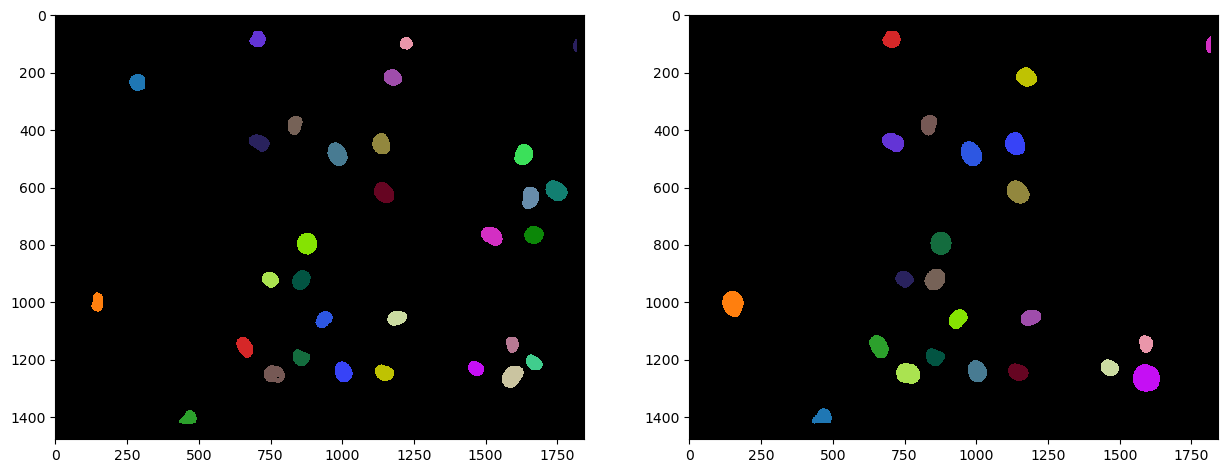The above shown label images have inside nuclei pixel intensity values that correspond to the number of the nucleus. In nucleus 1, all pixels have intensity 1. In nucleus 2, all pixels have intensity 2 and so on. Hence, from these label images, we can already determine the number of nuclei in both channels, by measuring the maximum intensity in the label images:

# determine maximum in both label images
number_of_nuclei_cy3 = nuclei_cy3.max()
number_of_nuclei_egfp = nuclei_egfp.max()

# print out result
print("Nuclei Cy3 positive:", number_of_nuclei_cy3)
print("Nuclei eGFP positive:", number_of_nuclei_egfp)

Nuclei Cy3 positive: 31.0
Nuclei eGFP positive: 23.0


Technically, we haven’t checked yet if all eGFP positive nuclei are also Cy3 positive. We can do this by determining how many eGFP positive nuclei are close by each individual Cy3 positive nucleus. Therefore, we need to set a maximum distance threshold allowing us to specify how far away centroids of nuclei are allowed to be.

maximum_distance = 15 # pixels

# draw a parametric map of cell counts
count_map = cle.proximal_other_labels_count_map(nuclei_cy3, nuclei_egfp)
cle.imshow(count_map, colorbar=True)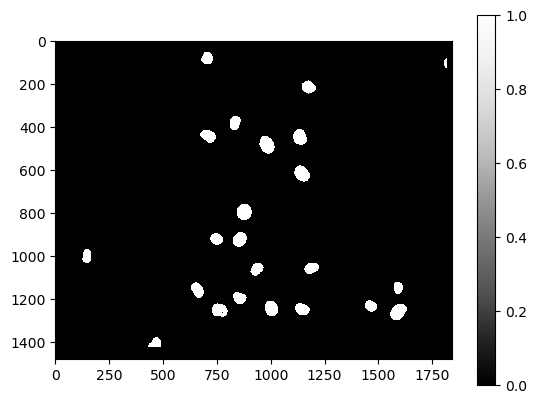The count_map is a parametric image. We can identify all the nuclei where the count value >= 1. These are all the Cy3-positive nuclei which have at least one eGFP-positive nucleus with a centroid distance <= 15 pixels.

double_positive_nuclei = cle.exclude_labels_with_map_values_out_of_range(
count_map,
nuclei_cy3,
minimum_value_range=1)

cle.imshow(double_positive_nuclei, labels=True)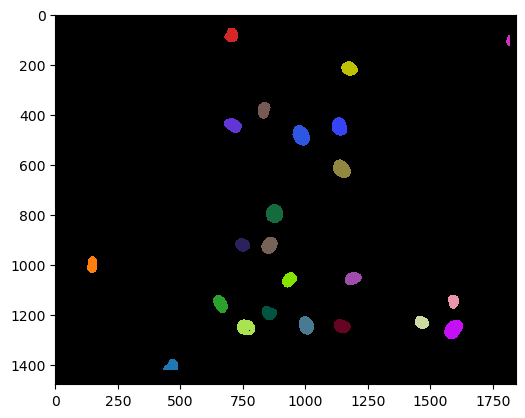And we can also count those similar to shown above:

number_of_double_positives = double_positive_nuclei.max()
print("Number of Cy3 positives that also express eGFP", number_of_double_positives)

Number of Cy3 positives that also express eGFP 23.0


## Visualization#

We can also use the outlines around cells which are double positive and visualize those on the original images of both channels.

# determine outlines
outlines = cle.detect_label_edges(double_positive_nuclei)

# add outlines to original images. As outlines have value 1,
# we need to multiply them to make them properly visible:
channel_0_with_outlines = cle.maximum_images(channel_0, outlines * channel_0.max())

# visualize result
cle.imshow(channel_0_with_outlines)

# let's zoom in
cle.imshow(channel_0_with_outlines.get()[400:800, 1000:1700])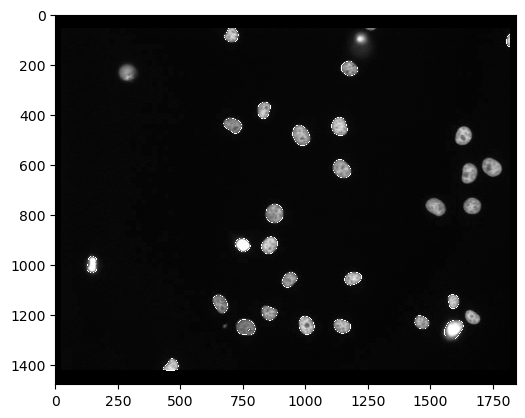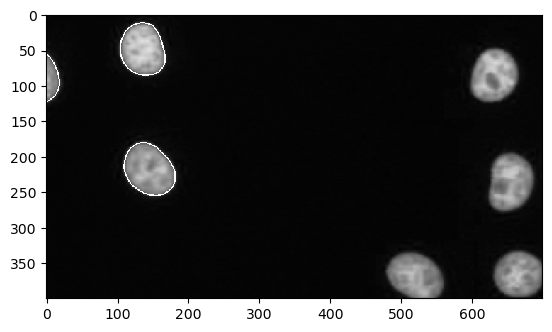For interactive visualization, we can also use napari:

# startup a viewer
import napari
viewer = napari.Viewer()

# add raw images in color to the viewer